The MI Procedure

Checking Convergence in MCMC

Subsections:

The theoretical convergence of the MCMC method has been explored under various conditions, as described in Schafer (1997, p. 70). However, in practice, verification of convergence is not a simple matter.

The parameters used in the imputation step for each iteration can be saved in an output data set with the OUTITER= option. These include the means, standard deviations, covariances, worst linear function, and observed-data LR statistics. You can then monitor the convergence in a single chain by displaying trace plots and autocorrelations for those parameter values (Schafer, 1997, p. 120). The trace and autocorrelation function plots for parameters such as variable means, covariances, and the worst linear function can be displayed by specifying the TIMEPLOT and ACFPLOT options, respectively.

You can apply the EM algorithm to a bootstrap sample to obtain overdispersed starting values for multiple chains (Gelman and Rubin, 1992). This provides a conservative estimate of the number of iterations needed before each imputation.

The next four subsections describe useful statistics and plots that can be used to check the convergence of the MCMC method.

LR Statistics

You can save the observed-data likelihood ratio (LR) statistic in each iteration with the LR option in the OUTITER= data set. The statistic is based on the observed-data likelihood with parameter values used in the iteration and the observed-data maximum likelihood derived from the EM algorithm.

In each iteration, the LR statistic is given by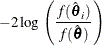whereis the observed-data maximum likelihood derived from the EM algorithm andis the observed-data likelihood forused in the iteration.

Similarly, you can also save the observed-data LR posterior mode statistic for each iteration with the LR_POST option. This statistic is based on the observed-data posterior density with parameter values used in each iteration and the observed-data posterior mode derived from the EM algorithm for posterior mode.

For large samples, these LR statistics tends to be approximatelydistributed with degrees of freedom equal to the dimension of(Schafer, 1997, p. 131). For example, with a large number of iterations, if the values of the LR statistic do not behave like a random sample from the describeddistribution, then there is evidence that the MCMC method has not converged.

Worst Linear Function of Parameters

The worst linear function (WLF) of parameters (Schafer, 1997, pp. 129–131) is a scalar function of parametersand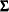that is "worst" in the sense that its function values converge most slowly among parameters in the MCMC method. The convergence of this function is evidence that other parameters are likely to converge as well.

For linear functions of parameters, a worst linear function ofhas the highest asymptotic rate of missing information. The function can be derived from the iterative values ofnear the posterior mode in the EM algorithm. That is, an estimated worst linear function ofiswhereis the posterior mode and the coefficients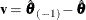are the difference between the estimated value ofone step prior to convergence and the converged value.

You can display the coefficients of the worst linear function,, by specifying the WLF option in the MCMC statement. You can save the function value from each iteration in an OUTITER= data set by specifying the WLF option within the OUTITER option. You can also display the worst linear function values from iterations in an autocorrelation plot or a trace plot by specifying WLF as an ACFPLOT or TIMEPLOT option, respectively.

Note that when the observed-data posterior is nearly normal, the WLF is one of the slowest functions to approach stationarity. When the posterior is not close to normal, other functions might take much longer than the WLF to converge, as described in Schafer (1997, p.130).

Trace Plot

A trace plot for a parameteris a scatter plot of successive parameter estimates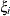against the iteration number i. The plot provides a simple way to examine the convergence behavior of the estimation algorithm for. Long-term trends in the plot indicate that successive iterations are highly correlated and that the series of iterations has not converged.

You can display trace plots for worst linear function, variable means, variable variances, and covariances of variables. You can also request logarithmic transformations for positive parameters in the plots with the LOG option. When a parameter value is less than or equal to zero, the value is not displayed in the corresponding plot.

By default, the MI procedure uses solid line segments to connect data points in a trace plot. You can use the CCONNECT=, LCONNECT=, and WCONNECT= options to change the color, line type, and width of the line segments, respectively. When WCONNECT=0 is specified, the data points are not connected, and the procedure uses the plus sign (+) as the plot symbol to display the points with a height of one (percentage screen unit) in a trace plot. You can use the SYMBOL=, CSYMBOL=, and HSYMBOL= options to change the shape, color, and height of the plot symbol, respectively.

By default, the plot title "Trace Plot" is displayed in a trace plot. You can request another title by using the TITLE= option in the TIMEPLOT option. When another title is also specified in a TITLE statement, this title is displayed as the main title and the plot title is displayed as a subtitle in the plot.

You can use options in the GOPTIONS statement to change the color and height of the title. See the chapter "The SAS/GRAPH Statements" in SAS/GRAPH: Reference for an illustration of title options. See Example 63.11 for a usage of the trace plot.

Autocorrelation Function Plot

To examine relationships of successive parameter estimates, the autocorrelation function (ACF) can be used. For a stationary series,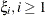, in trace data, the autocorrelation function at lag k is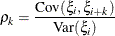The sample kth order autocorrelation is computed asYou can display autocorrelation function plots for the worst linear function, variable means, variable variances, and covariances of variables. You can also request logarithmic transformations for parameters in the plots with the LOG option. When a parameter has values less than or equal to zero, the corresponding plot is not created.

You specify the maximum number of lags of the series with the NLAG= option. The autocorrelations at each lag less than or equal to the specified lag are displayed in the graph. In addition, the plot also displays approximate 95% confidence limits for the autocorrelations. At lag k, the confidence limits indicate a set of approximate 95% critical values for testing the hypothesis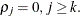By default, the MI procedure uses the star (*) as the plot symbol to display the points with a height of one (percentage screen unit) in the plot, a solid line to display the reference line of zero autocorrelation, vertical line segments to connect autocorrelations to the reference line, and a pair of dashed lines to display approximately 95% confidence limits for the autocorrelations.

You can use the SYMBOL=, CSYMBOL=, and HSYMBOL= options to change the shape, color, and height of the plot symbol, respectively, and the CNEEDLES= and WNEEDLES= options to change the color and width of the needles, respectively. You can also use the LREF=, CREF=, and WREF= options to change the line type, color, and width of the reference line, respectively. Similarly, you can use the LCONF=, CCONF=, and WCONF= options to change the line type, color, and width of the confidence limits, respectively.

By default, the plot title "Autocorrelation Plot" is displayed in a autocorrelation function plot. You can request another title by using the TITLE= option within the ACFPLOT option. When another title is also specified in a TITLE statement, this title is displayed as the main title and the plot title is displayed as a subtitle in the plot.

You can use options in the GOPTIONS statement to change the color and height of the title. See the chapter "The SAS/GRAPH Statements" in SAS/GRAPH: Reference for a description of title options. See Example 63.8 for an illustration of the autocorrelation function plot.